#### Data Structure and Algorithms with JS - Part 0

DSA
JavaScript

Data structures are an essential aspect of computer science and programming. They provide a way to organize and store data in a computer so that it can be accessed and modified efficiently. In this blog, we will cover some basic concepts and common data structures that you should know as a programmer.

#### What is a data structure?

A data structure is a way of organizing and storing data in a computer so that it can be accessed and modified efficiently. There are many different types of data structures, each with its own set of benefits and drawbacks.

Some common data structures include arrays, linked lists, stacks, queues, trees, and graphs.

##### Arrays

An array is a linear data structure that stores a fixed-size sequence of elements of the same data type. The elements in an array are stored in contiguous memory locations and can be accessed using an index. Arrays are efficient for accessing and modifying elements, but they are not very flexible when it comes to inserting and deleting elements.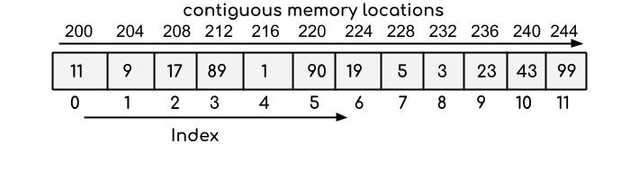A linked list is a linear data structure that consists of a sequence of nodes. Each node contains a value and a reference (or pointer) to the next node in the list. Linked lists are flexible because they allow you to insert and delete elements easily, but they are not as efficient as arrays for accessing and modifying elements.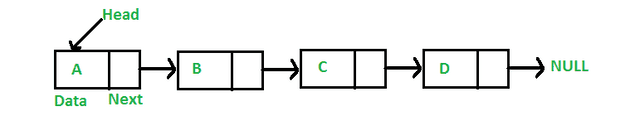##### Stacks

A stack is a linear data structure that follows the last-in, first-out (LIFO) principle. It has two main operations: push and pop. Push adds an element to the top of the stack, and pop removes the element from the top of the stack. Stacks are often used to implement undo/redo functionality and to evaluate expressions.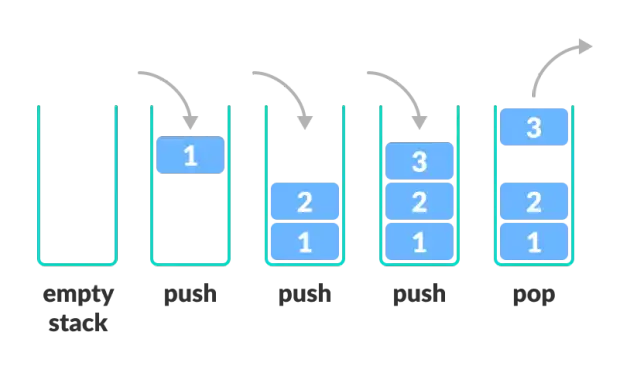##### Queues

A queue is a linear data structure that follows the first-in, first-out (FIFO) principle. It has two main operations: enqueue and dequeue. Enqueue adds an element to the end of the queue, and dequeue removes the element from the front of the queue. Queues are often used to implement task scheduling and buffering.##### Trees

A tree is a non-linear data structure that consists of nodes organized into a hierarchy. The top node is called the root, and the nodes below it are called child nodes. Each child node may have its own child nodes, forming a sub-tree. Trees are often used to represent hierarchical relationships and to perform operations such as searching and sorting.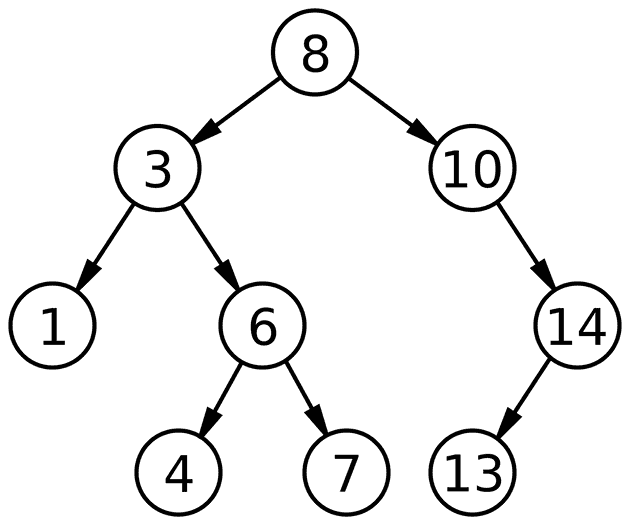##### Graphs

A graph is a non-linear data structure that consists of a set of vertices (or nodes) and a set of edges that connect the vertices. Graphs can be directed (where the edges have a direction) or undirected (where the edges have no direction). They are often used to represent relationships and connections between data.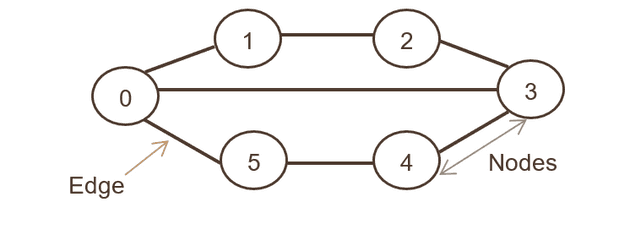#### Choosing the right data structure

When choosing a data structure, it’s important to consider the operations that you will be performing on the data and the efficiency of each data structure for those operations. For example, if you need to access and modify elements frequently, an array might be a better choice than a linked list. On the other hand, if you need to insert and delete elements frequently, a linked list might be a better choice.

#### What is an Algorithm?

As a computer programmer, it is important to understand the basics of algorithms and time space complexity. An algorithm is a set of steps that can be followed to solve a problem. It is the foundation of computer programming and is used to create software, applications, and websites.

One important aspect of algorithms is their time and space complexity, which measures how efficient an algorithm is in terms of the resources it uses. Time complexity refers to the amount of time an algorithm takes to complete its task, while space complexity refers to the amount of memory or storage space it requires.

##### What is Complexity Notation!?

One way to measure the efficiency of an algorithm is through the use of Big O notation. Big O notation is a way to describe the upper bound of an algorithm’s time complexity. It is typically used to describe the worst case scenario, or the maximum amount of time it will take for the algorithm to run.

For example, if an algorithm has a time complexity of O(n), this means that the time it takes to run will increase linearly with the size of the input (n). An algorithm with a time complexity of O(n^2) means that the time it takes to run will increase with the square of the size of the input.

Other common notations for time complexity include Omega notation (Ω), which describes the lower bound of an algorithm’s time complexity, and Theta notation (Θ), which describes both the upper and lower bounds of an algorithm’s time complexity.

Here is a table comparing the different notations:

Notation Meaning
O Upper bound
Ω Lower bound
Θ Both upper and lower bounds

There are several different types of time and space complexity cases.

###### The most common ones are:

O(n) - This type represents the linear time complexity of an algorithm, where n is the size of the input data. An algorithm with an O(n) time complexity means that the time it takes to complete the task increases linearly with the size of the input data.

O(1) - O(1) time complexity is considered the best possible time complexity. This represents a constant time complexity, meaning that the time it takes to complete the task does not depend on the size of the input data.

O(log n) - This type represents a logarithmic time complexity, meaning that the time it takes to complete the task increases logarithmically with the size of the input data.

O(n log n) - This type represents a time complexity that is a combination of linear and logarithmic.

O(n^2) - This type represents a quadratic time complexity, meaning that the time it takes to complete the task increases exponentially with the size of the input data.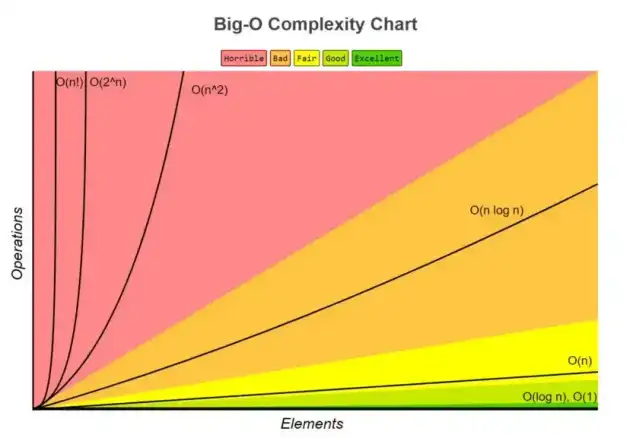Big-O Complexity Chart: http://bigocheatsheet.com/

In general, we want to choose algorithms with the lowest possible time complexity. However, it’s important to keep in mind that time complexity is just one factor to consider when choosing an algorithm. Other factors, such as the simplicity of the algorithm and the amount of space it requires, may also be important considerations.

Overall, understanding algorithm and time space complexity is crucial for choosing the most efficient solution to a problem. By using the different types and understanding their meanings, we can better evaluate the efficiency of different algorithms and choose the one that best fits our needs.Hey there! This is Vishal Sharma. I reside and work at Gurgaon, India. I am a Software Engineer and primarily works with JavaScript, ReactJS and NodeJS.Welcome to my Javascript tech blog! Here, you'll find the latest news and updates in the world of Javascript, as well as tutorials and resources to help you improve your coding skills. From learning the basics of Javascript to advanced concepts like object-oriented programming, I post something for developers of all levels. Whether you're just starting out or you're a seasoned pro, you'll find valuable insights and information on our blog. So stay up-to-date with the latest in Javascript technology by bookmarking this page and checking back often.
Thank you for visiting!